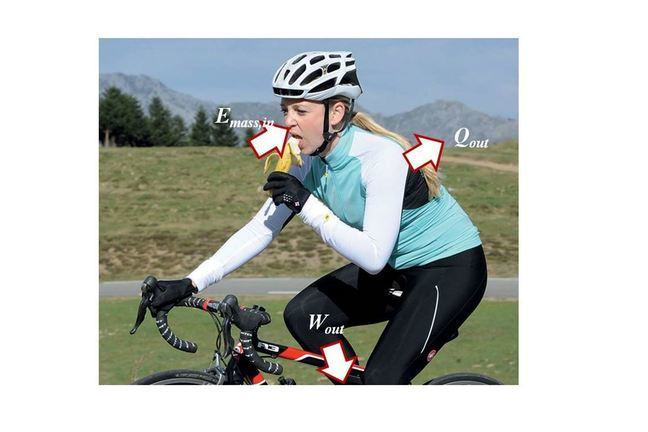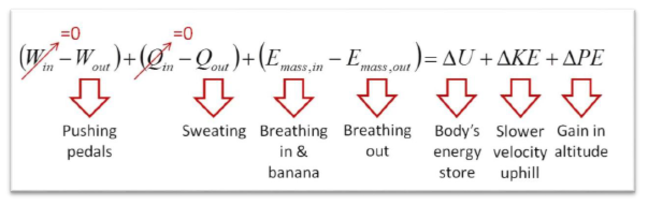## Want to keep learning?

This content is taken from the University of Liverpool's online course, Energy: Thermodynamics in Everyday Life. Join the course to learn more.
1.6

## University of Liverpool# First law of thermodynamics

The first law of thermodynamics states that energy is always conserved…

i.e. it can neither be created nor destroyed. In thermodynamics, we are interested in processes that involve transforming energy from one form to another which often involves work and heat. We usually call the part of the universe that we are interested in, ‘the system’ and everything else ‘the surroundings’. More formally, ‘a thermodynamic system is a well-defined quantity of matter and which can exchange energy with its environment’1 . For a system undergoing a process, we can express the first law as the sum of the energy flows across its boundary is equal to the change in energy content of the system, i.e.

E in - Eout = $$\Delta$$Esystem 

where $$\Delta$$Esystem is the change in the energy of the system and E in and Eout are the energy flows in and out respectively. Energy can flow across the boundary as work done either to the system or by the system, as heat transfer in or out of the system or it can be carried in a flow of matter across the boundary. Hence,

E in - Eout = (Win - Wout) + (Qin - Qout) + (E mass,in - Emass,out ) 

where Emass, Q and W are the energy carried by matter, heat transfer and work moving in and out of the system, as indicated by the subscripts. The common ways that we can store energy in a system are as internal energy, U and as a result of its position and motion, i.e. potential energy, PE and kinetic energy, KE respectively. Hence,

$$\Delta$$Esystem = $$\Delta$$U + $$\Delta$$KE + $$\Delta$$PE 

We can substitute equations (2) and (3) into equation (1) to arrive at the classical expression of the first law of thermodynamics for system:

(Win - Wout) + (Qin - Qout) + (E mass,in - Emass,out ) = $$\Delta$$U + $$\Delta$$KE + $$\Delta$$PE 

I have highlighted this equation in bold because it is one of the most powerful equations that we will encounter in this course. This expression of the first law of thermodynamics allows us to analyse a large number of systems and make predictions about their behaviour. Let’s start with an everyday example of riding a bicycle uphill while eating a banana. I am going to define the ‘system’ as the person on the bike, i.e. just the cyclist not the bike. The cyclist is going uphill and so she will be doing work pushing the pedals around, i.e. there is work output from the system but I can’t identify any work inputs (Win = 0).

Let’s assume that she is cycling in the UK, in which case the cyclist will be hotter than the surroundings and so we can expect them to lose heat to the surroundings and to gain none (Qin = 0). The cyclist will be breathing air in and out so that there is energy being carried in and out with each breath. She is also eating a banana so energy is entering the system every time she swallows a bite.

All of these energy flows across the system boundary will change the total energy content of the system which will be made up of the energy stored in the cyclist’s body, U; the loss of kinetic energy since most cyclists get slower and slower going uphill and the gain in potential energy due to increase in height above sea level.Figure 1: Annotated version of equation (4) for a cyclist eating a banana while cycling uphill.

If you feel that you have mastered the content of this article then watch the worked example in 1.7.

Please note refreshing the page will remove any ‘Maths Processing Error’ notifications and load the script correctly if you encounter problems when first viewing this article. Thank you to Ged Langosz for highlighting this in the first run of the course.

1 Thess, A., The Entropy Principle: Thermodynamics for the unsatisfied, Berlin: Springer-Verlag, 2011.